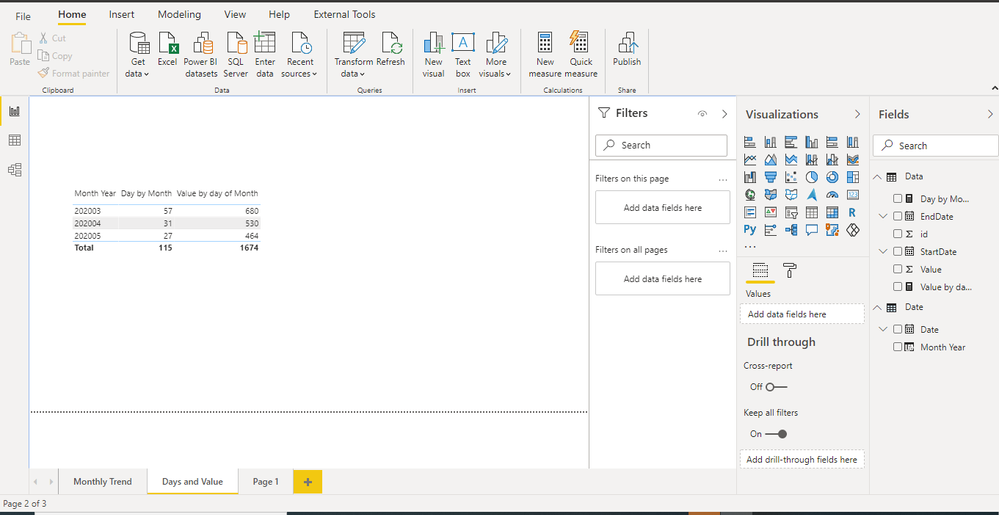cancel
Showing results for
Did you mean:## How to divide/distribute values between start date or end date or count days across months/days

Objective:

How to display data month wise, when values are provided between a start and end dates.

Solution:

Step1: We have got the following data.Step 2: Created a date table with Month year as one of the columns

``````Date = CALENDAR( date(2019,01,01),date(2021,01,01))
Column:
Month Year = FORMAT('Date'[Date],"YYYYMM")``````Now we need to display this data by dates or month. For this, we need to split data by date.While the approach can be to create a table using a cross join and filter. We will this approach in our measures.

``````Day by Month = CALCULATE(countx(SUMMARIZE(filter(CROSSJOIN('Data','Date'),'Date'[Date] >= 'Data'[StartDate] && 'Date'[Date]<= 'Data'[EndDate]),'Data'[id],'Date'[Date]),'Date'[Date]))

Value by day of Month = CALCULATE(SUMX(SUMMARIZE(filter(CROSSJOIN('Data','Date'),'Date'[Date] >= 'Data'[StartDate] && 'Date'[Date]<= 'Data'[EndDate]),'Data'[id],'Date'[Date],Data[Value],Data[StartDate],Data[EndDate]),DIVIDE(Data[Value],DATEDIFF(Data[StartDate],Data[EndDate],day)+1)))
``````The file can found at : https://github.com/amitchandakpbi/powerbi/blob/main/DistributebetweenDays.pbix

You can get all my posts at https://community.powerbi.com/t5/Data-Stories-Gallery/Blog-Analysis/m-p/1265567#M4403